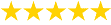# Electricity Cost Calculator

This power cost calculator estimates the energy usage and annual running cost of any appliance. All you need to enter is:1) How much power the appliance draws in Watts. You can find this on most compliance plates, as pictured here. For better accuracy use a plug-in power meter or energy monitor (for large appliances).

2) Your electricity tariff in cents per kWh. You can find this on your electricity bill. Enter 25 if you don't have a bill handy.

3) An estimate of how often the appliance is used.

## Appliance Electricity Cost Calculator:

### Tips When Calculating Appliance Running Costs

Appliances with a fairly constant power draw are ideal for use with this electricity cost calculator. Examples include: lights, heaters, pumps, motors, and fans.

Some appliances can be switched on 24/7 but only draw power for some of this time. For example, a refrigerator's compressor may only draw power for 8 to 12 hours per day. For these appliances you can either make an estimate based on your observations, or use a power meter.

For other appliances, the power usage can vary a lot. For example, a dishwasher may use 2400W when heating, 50W when pumping water, and 5W in standby. Estimating an 'average power' for these appliances can be tricky. Any appliance which goes through a 'cycle' like this is best measured with a power meter

Strictly speaking, there are 52 weeks and 1 day in a non-leap year. For simplicity, our calculator ignores this and bases a year on 52 weeks (364 days) only. We think it's easier to calculate energy usage in terms of 'days per week' and 'weeks per year' rather than say 'days per year' or 'hours or year'. For example: many devices operate for 5 days per week, and many people take a holiday for 2 weeks per year.

### Example Energy Cost Calculations

Here are some example calculations for common appliances. Enter them above and change the inputs to see the impact. These examples assume an electricity tariff of 25 cents per kWh.

 Appliance Usage Annual Cost Pool Pump (1200W) 8h/day \$873.60 Fridge / Freezer (140W) 10h/day \$127.40 TV (100W on) 5h/day \$45.50 TV (5W in standby) 19h/day \$8.65 Ducted AC (4500W) 6h/day, 24wk/yr \$1,134 Clothes Dryer (2400W) 6h/wk, 50wk/yr \$180

### How to Calculate Electricity Usage Costs

The above power calculator uses a simple formula to calculate annual energy usage and costs. Here's how it works:

Electricity Usage Per Year = (Watts / 1000) * Hours/Day * Days/Week * Weeks/Year = kWh/year

Electricity Cost Per Year = kWh/year * (cents/kWh / 100) = \$/year

### Got a Question? We're Here to Help!Read Our Customer Reviews!